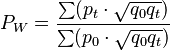# Difference between revisions of "Walsh price index"$P_W = \frac{\sum(p_t\cdot \sqrt{q_0 q_t})}{\sum(p_0\cdot \sqrt{q_0 q_t})}$